Share

# Selina solutions for Concise Mathematics Class 10 ICSE chapter 22 - Height and Distances [Latest edition]

Course
Textbook page

#### Chapters## Chapter 22: Height and Distances

Exercise 22(A)Exercise 22(B)Exercise 22(C)

#### Selina solutions for Concise Mathematics Class 10 ICSE Chapter 22 Height and Distances Exercise Exercise 22(A) [Pages 336 - 337]

Exercise 22(A) | Q 1 | Page 336

The height of a tree is √3 times the length of its shadow. Find the angle of elevation of the sun.

Exercise 22(A) | Q 2 | Page 336

The angle of elevation of the top of a tower from a point on the ground and at a distance of 160 m from its foot, is found to be 60o. Find the height of the tower.

Exercise 22(A) | Q 3 | Page 336

A ladder is placed along a wall such that its upper end is resting against a vertical wall. The foot of the ladder is 2.4 m from the wall and the ladder is making an angle of 68o with the ground. Find the height, upto which the ladder reaches.

Exercise 22(A) | Q 4 | Page 336

Two persons are standing on the opposite sides of a tower. They observe the angles of elevation of the top of the tower to be 30o and 38o respectively. Find the distance between them, if the height of the tower is 50 m.

Exercise 22(A) | Q 5 | Page 337

A kite is attached to a string. Find the length of the string, when the height of the kite is 60 m and the string makes an angle 30o with the ground

Exercise 22(A) | Q 6 | Page 337

A boy, 1.6 m tall, is 20 m away from a tower and observes the angle of elevation of the top of the tower to be (i) 45o, (ii) 60o. Find the height of the tower in each case.

Exercise 22(A) | Q 7 | Page 337

The upper part of a tree, broken over by the wind, makes an angle of 45o with the ground and the distance from the root to the point where the top of the tree touches the ground is 15 m. What was the height of the tree before it was broken?

Exercise 22(A) | Q 8 | Page 337

The angle of elevation of the top of an unfinished tower at a point distance 80 m from its base is 30°. How much higher must the tower be raised so that its angle of elevation at the same point may be 60°?

Exercise 22(A) | Q 9 | Page 337

At a particular time, when the sun’s altitude is 30o, the length of the shadow of a vertical tower is 45 m. Calculate (i) the length of the tower. (ii) the length of the shadow of the same tower, when the sun’s altitude is (a) 45° (b) 60°

Exercise 22(A) | Q 10 | Page 337

Two vertical poles are on either side of a road. A 30 m long ladder is placed between the two poles. When the ladder rests against one pole, it makes angle 32o24′ with the pole and when it is turned to rest against another pole, it makes angle 32o24′ with the road. Calculate the width of the road.

Exercise 22(A) | Q 11 | Page 337

Two climbers are at points A and B on a vertical cliff face. To an observer C, 40m from the foot of the cliff, on the level ground, A is at an elevation of 48o and B of 57°. What is the distance between the climbers?

Exercise 22(A) | Q 12 | Page 337

A man stands 9 m away from a flag-pole. He observes that angle of elevation of the top of the pole is 28o and the angle of depression of the bottom of the pole is 13o. Calculate the height of the pole.

Exercise 22(A) | Q 13 | Page 337

From the top of a cliff 92 m high, the angle of depression of a buoy is 20o. Calculate, to the nearest metre, the distance of the buoy from the foot of the cliff.

#### Selina solutions for Concise Mathematics Class 10 ICSE Chapter 22 Height and Distances Exercise Exercise 22(B) [Pages 341 - 342]

Exercise 22(B) | Q 1 | Page 341

In the figure, given below, it is given that AB is perpandiculer to BD  and is of length X metres. DC = 30m .∠ ADB = 30º and  ∠ ACB = 45°. Without using tables, find X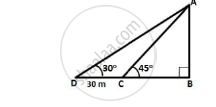Exercise 22(B) | Q 2 | Page 341

Find the height of a tree when it is found that on walking away from it 20 m, in a horizontal line through its base, the elevation of its top changes from 60o to 30o.

Exercise 22(B) | Q 3 | Page 341

Find the height of a building, when it is found that on walking towards it 40 m in a horizontal line through its base the angular elevation of its top changes from 30o to 45o.

Exercise 22(B) | Q 4 | Page 341

From the top of a light house 100 m high, the angles of depression of two ships are observed as 48o and 36o respectively. Find the distance between the two ships(in the nearest metre) if: (i) the ships are on the same side of the light house. (ii) the ships are on the opposite sides of the light house.

Exercise 22(B) | Q 5 | Page 341

Two pillars of equal heights stand on either side of a roadway, which is 150 m wide. At a point in the roadway between the pillars the elevations of the tops of the pillars are 60o and 30o ; find the height of the pillars and the position of the point.

Exercise 22(B) | Q 6 | Page 341

From the figure , given below , calculate the lenth of CD .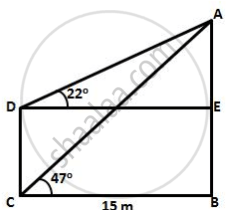Exercise 22(B) | Q 7 | Page 341

The angle of elevation of the top of a tower is observed to be 60°. At a point, 30 m vertically above the first point of observation, the elevation is found to be 45°. Find: (i) the height of the tower, (ii) its horizontal distance from the points of observation.

Exercise 22(B) | Q 8 | Page 341

From the top of a cliff, 60 metres high, the angles of depression of the top and bottom of a tower are observed to be 30o and 60o. Find the height of the tower.

Exercise 22(B) | Q 9 | Page 341

A man on a cliff observes a boat, at an angle of depression 30°, which is sailing towards the shore to the point immediately beneath him. Three minutes later, the angle of depression of the boat is found to be 60°. Assuming that the boat sails at a uniform speed, determine:

(i) how much more time it will take to reach the shore.
(ii) the speed of the boat in metre per second, if the height of the cliff is 500 m.

Exercise 22(B) | Q 10 | Page 341

A man in a boat rowing away from a lighthouse 150 m high, takes 2 minutes to change the angle of elevation of the top of the lighthouse from 60o to 45o. Find the speed of the boat.

Exercise 22(B) | Q 11 | Page 341

A person standing on the bank of a river observes that the angle of elevation of the top of a tree standing on the opposite bank is 60o. When he moves 40 m away from the bank, he finds the angle of elevation to be 30°. Find: (i) the height of the tree, correct to 2 decimal places, (ii) the width of the river.

Exercise 22(B) | Q 12 | Page 342

The horizontal distance between two towers is 75 m and the angular depression of the top of the first tower as seen from the top of the second, which is 160 m high, is 45°. Find the height of the first tower.

Exercise 22(B) | Q 13 | Page 342

The length of the shadow of a tower standing on level plane is found to be 2y metres longer when the sun’s altitude is 30o than when it was 45°. Prove that the height of the tower is y(√3 + 1) metres.

Exercise 22(B) | Q 14 | Page 342

An aeroplane flying horizontally 1 km above the ground and going away from the observer is observed at an elevation of 60°. After 10 seconds, its elevation is observed to be 30°; find the uniform speed of the aeroplane in km per hour.

Exercise 22(B) | Q 15 | Page 342

From the top of a hill, the angles of depression of two consecutive kilometer stones, due east, are found to be 30° and 45° respectively. Find the distances of the two stones from the foot of the hill.

#### Selina solutions for Concise Mathematics Class 10 ICSE Chapter 22 Height and Distances Exercise Exercise 22(C) [Pages 2 - 343]

Exercise 22(C) | Q 1.1 | Page 342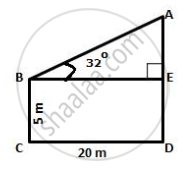Exercise 22(C) | Q 1.2 | Page 342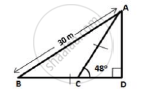Exercise 22(C) | Q 2 | Page 2

In the following diagram , AB is a floor- board : PQRS is a cubical box with each edge = 1m and ∠B = 60 °. Calculate the lenght of the board AB.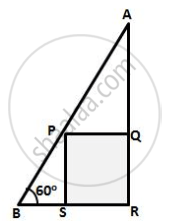Exercise 22(C) | Q 3 | Page 342

Calculate BC .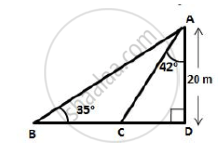Exercise 22(C) | Q 4 | Page 342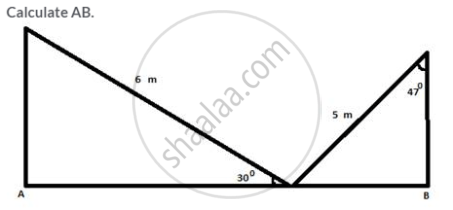Exercise 22(C) | Q 5 | Page 342

The radius of a circle is given as 15 cm and chord AB subtends an angle of 131o at the centre C of the circle. Using trigonometry, calculate:

(i) the length of AB;

(ii) the distance of AB from the centre C.

Exercise 22(C) | Q 6 | Page 342

At a point on level ground, the angle of elevation of a vertical tower is found to be such that its tangent is 5/12. On walking 192 metres towards the tower, the tangent of the angle is found to be 3/4. Find the height of the tower.

Exercise 22(C) | Q 7 | Page 342

A vertical tower stand on a horizontel plane and is surmounted by a vertical flagstaff of height h metre. At a point on the plane, the angle of elevation of the bottom of the flagstaff is ∝  and at the  top of the flagstaff is β . prove that the height of the tower is

"h   tan  ∝ "/"tan  β - tan  ∝"

Exercise 22(C) | Q 8 | Page 342

With reference to the given figure, a man stands on the ground at point A, which is on the same horizontal plane as B, the foot of the vertical pole BC. The height of the pole is 10 m. The man's eye s 2 m above the ground. He observes the angle of elevation of C, the top of the pole, as xo , where tan xo =2/5.
Calculate :
(i) the distance AB in metres.
(ii) angle of elevation of the top of the pole when he is standing 15 metres from the pole. Give your answer to the nearest degree.

Exercise 22(C) | Q 9 | Page 343

The angles of elevation of the top of a tower from two points on the ground at distances a and b metres from the base of the tower and in the same line are complementary. Prove that the height of the tower is √ab metre.

Exercise 22(C) | Q 10 | Page 343

From a window A , 10 m above the ground the angle of elevation of the top C of a tower is X °, Where tan x ° = 5/2  and the angle of depression of the foot D of the tower is Y° , Where tan y ° = 1/4.  calculate the height CD of the tower in metres .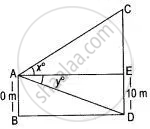Exercise 22(C) | Q 11 | Page 343

A vertical tower is 20 m high. A man standing at some distance from the tower knows that the cosine of the angle of elevation of the top of the tower is 0.53. How far is he standing from the foot of the tower?

Exercise 22(C) | Q 12 | Page 343

A man standing on the bank of a river observes that the angle of elevation of a tree on the opposite bank is 60°. When he moves 50 m away from the bank, he finds the angle of elevation to be 30°. Calculate:

(i) the width of the river;

(ii) the height of the tree.

Exercise 22(C) | Q 13 | Page 343

A 20 m high vertical pole and a vertical tower are on the same level ground in such a way that the angle of elevation of the top of the tower, as seen from the foot of the pole is 60° and the angle of elevation of the top of the pole, as seen from the foot of the tower is 30°. Find:

(i) the height of the tower ;

(ii) the horizontal distance between the pole and the tower.

Exercise 22(C) | Q 14.1 | Page 343

A vertical pole and a vertical tower are on the same level ground in such a way that from the top of the pole, the angle of elevation of the top of the tower is 60o and the angle of depression of the bottom of the tower is 30o. Find: the height of the tower, if the height of the pole is 20 m.

Exercise 22(C) | Q 14.2 | Page 343

A vertical pole and a vertical tower are on the same level ground in such a way that from the top of the pole, the angle of elevation of the top of the tower is 60o and the angle of depression of the bottom of the tower is 30o. Find: the height of the pole, if the height of the tower is 75 m.

Exercise 22(C) | Q 15 | Page 343

From a point, 36 m above the surface of a lake, the angle of elevation of a bird is observed to be 30o and the angle of depression of its image in the water of the lake is observed to be 60o. Find the actual height of the bird above the surface of the lake.

Exercise 22(C) | Q 16 | Page 343

A man observes the angle of elevation of the top of a building to be 30°. He walks towards it in a horizontal line through its base. On covering 60 m, the angle of elevation changes to 60°. Find the height of the building correct to the nearest metre.

Exercise 22(C) | Q 17 | Page 343

As observed from the top of a 80 m tall lighthouse, the angles of depression of two ships, on the same side of a light house in a horizontal line with its base, are 30° and 40° respectively. Find the distance between the two ships. Give your answer corrected to the nearest metre.

Exercise 22(C) | Q 18 | Page 343

In the given figure, from the top of a building AB = 60 m hight, the angle of depression of the top and bottom of a vertical lamp post CD are observed to be 30 ° and 60 ° respectively. find :

1) the horizental  distance between AB and CD ;

2) the height of the lamp post.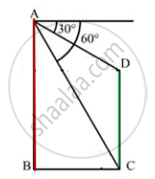Exercise 22(C) | Q 19 | Page 343

An aeroplane, at an altitude of 250 m, observes the angles of depression of two boats on the opposite banks of a river to be 45° and 60° respectively. Find the width of the river. Write the answer correct to the nearest whole number.

Exercise 22(C) | Q 20 | Page 343

The horizontal dist6ance between two towers is 120 m. The angle of elevation of the top and angle of depression of the bottom of the first tower as observed from the top of the second i9s 30 ° and 24 ° respectively. find the height of the two towers. give your answers correct to 3 significant figures.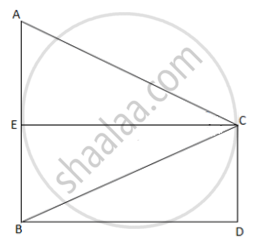Exercise 22(C) | Q 21 | Page 343

The angles of depression of two ships A and B as observed from the top of a lighthouse 60 m high are 60° and 45° respectively. If the two ships are on the opposite sides of the lighthouse, find the distance between the two ships, Give your answer correct to the nearest whole number.

## Chapter 22: Height and Distances

Exercise 22(A)Exercise 22(B)Exercise 22(C)## Selina solutions for Concise Mathematics Class 10 ICSE chapter 22 - Height and Distances

Selina solutions for Concise Mathematics Class 10 ICSE chapter 22 (Height and Distances) include all questions with solution and detail explanation. This will clear students doubts about any question and improve application skills while preparing for board exams. The detailed, step-by-step solutions will help you understand the concepts better and clear your confusions, if any. Shaalaa.com has the CISCE Concise Mathematics Class 10 ICSE solutions in a manner that help students grasp basic concepts better and faster.

Further, we at Shaalaa.com provide such solutions so that students can prepare for written exams. Selina textbook solutions can be a core help for self-study and acts as a perfect self-help guidance for students.

Concepts covered in Concise Mathematics Class 10 ICSE chapter 22 Height and Distances are Trigonometric Ratios of Complementary Angles, Trigonometric Identities, Heights and Distances - Solving 2-D Problems Involving Angles of Elevation and Depression Using Trigonometric Tables, Trigonometry Problems and Solutions.

Using Selina Class 10 solutions Height and Distances exercise by students are an easy way to prepare for the exams, as they involve solutions arranged chapter-wise also page wise. The questions involved in Selina Solutions are important questions that can be asked in the final exam. Maximum students of CISCE Class 10 prefer Selina Textbook Solutions to score more in exam.

Get the free view of chapter 22 Height and Distances Class 10 extra questions for Concise Mathematics Class 10 ICSE and can use Shaalaa.com to keep it handy for your exam preparation